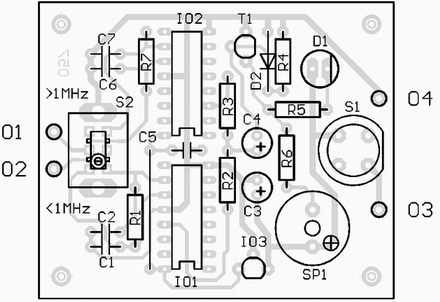# Crystal Tester Electronic Schematics > Test and measurement > Crystal Tester

This circuit enables you to test quartz resonators at the range values from 32kHz to 24MHz. Confirmation of good state of quartz resonator is done by diode signalling LED and acoustic signal. Switch S2 enables change of range .

Parts List

R1,R7 = 1 M
R2,R3 = 5,6 k
R4 = 220 K
R5 = 1 k
R6 = 220
C1,C2 = 220 pF
C3,C4 = 10 F/25V RSM
C5 = 100 nF
C6,C7 = 33 pF
D1 = LED 5 mm, yellow
D2 = 1N4148
T1 = BC547C
IO1 = 4060
IO2 = 74HC4060
IO3 = 78L05
S1 = push button (normally open)
S2 = Two-positional switchTitle: Crystal Tester
electronic circuit
Source: www.elektroda.pl
Published on: 2005-02-10
Print version: